# Finance Calculator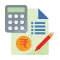## EMI Calculator

Calculate your personal loan, home loan, or car loan EMI with BankBazaar EMI Calculator.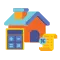## Home Loan EMI Calculator

Calculate your home loan EMI with our Home Loan Calculator.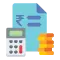## Personal Loan EMI Calculator

Calculate your personal loan EMI with our Personal Loan Calculator.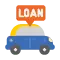## Car Loan EMI Calculator

Calculate your car loan EMI with our Car Loan EMI Calculator.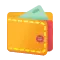## FD Calculator

Calculate your FD (Fixed Deposit) returns easily with our FD Calculator.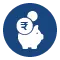## RD Interest Calculator

Calculate your RD (Recurring Deposit) returns easily with our RD Calculator.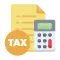## Income Tax Calculator

Calculate your income tax slab-based payout with the help of our Income Tax Calculator.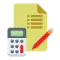## HRA Calculator

Calculate your HRA (House Rent Allowance) conveniently with our HRA Calculator.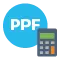## PPF Calculator

Calculate your PPF (Public Provident Fund) returns conveniently with our PPF Calculator.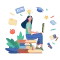## Sukanya Samriddhi Yojana Calculator

Calculate returns on your investment toward Sukanya Samriddhi Yojana (SSY).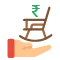## NPS Calculator

Calculate your NPS (National Pension Scheme) returns seamlessly with Bankbazaar NPS Calculator.

reTH65gcmBgCJ7k